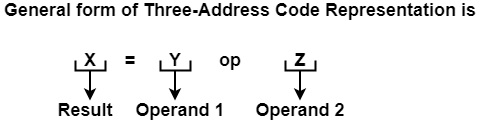# What is Three Address Code?

The three-address code is a sequence of statements of the form A−=B op C, where A, B, C are either programmer-defined names, constants, or compiler-generated temporary names, the op represents an operator that can be constant or floatingpoint arithmetic operators or a Boolean valued data or a logical operator. The reason for the name “three address code” is that each statement generally includes three addresses, two for the operands, and one for the result.

In the three-address code, atmost three addresses are define any statement. Two addresses for operand & one for the result.Hence, op is an operator.

An only a single operation is allowed at a time at the right side of the expression.

Example− Expression a = b + c + d can be converted into the following Three Address Code.

t1 = b + c

t2 = t1 + d

a = t2

where t1 and t2 are temporary variables generated by the compiler. Most of the time a statement includes less than three references, but it is still known as a threeaddress statement.

Following are the various types of three address statements −

• Assignment− The three types of Assignment statements are

x = y op z, op is a binary operator with y, z as operands.

x = op y, op is a unary operator

x = y, values of y is assigned to x.

• Unconditional Jumps− Unconditional Jump is of the form goto L, L being a label.

Control flow to three address statements labeled at L.

• Conditional Jump − Condition Jump is of the form

if x relop y goto L

Here relop can be <, >, <=, >=. If the condition is true, then it can execute three address statements at Label L else statement following if statement will be executed.

• Array Statements

x = y[i], value of ithlocation of array y is assigned to x.

x[i] = y, the value of y is assigned to ithlocation of array x.

• Address & Pointer Assignments − Languages like Pascal & C allow pointer assignments.

x = & y, the address of y is assigned to x.

x = * y, the content of location pointed to by y is assigned to x.

*x=y, Finally sets the r-value of the object pointed to by x to the r-value of y.

• Procedure call/Return − A call to the procedure P(x1, x2 … . . xn) with the parameters x1, x2 … . . xn is written as

param x1

param x2

………..

param xn

call p, n

Here param refers to the parameter, & call p, n will call procedure p with n arguments.